# ggmatrix

## Introduction

`ggmatrix` is a function for managing multiple plots in a matrix-like layout. It was designed to adapt to any number of columns and rows. This allows for very customized plot matrices.

## Generic Example

The examples below use plots labeled 1 to 6 to distinguish where the plots are being placed.

``````plotList <- list()
for (i in 1:6) {
plotList[[i]] <- ggally_text(paste("Plot #", i, sep = ""))
}

# bare minimum of plotList, nrow, and ncol
pm <- ggmatrix(plotList, 2, 3)
pm``````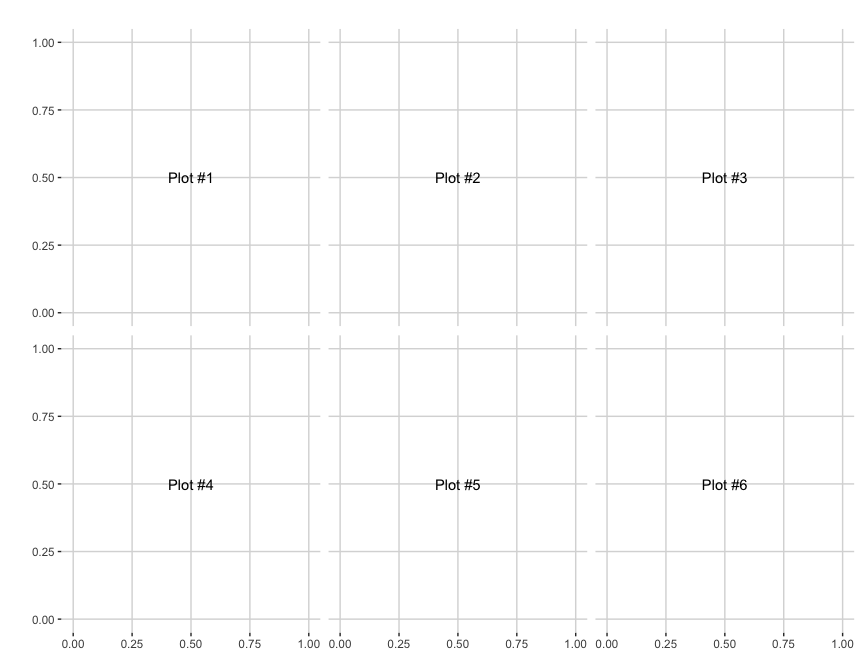``````# provide more information
pm <- ggmatrix(
plotList,
nrow = 2, ncol = 3,
xAxisLabels = c("A", "B", "C"),
yAxisLabels = c("D", "E"),
title = "Matrix Title"
)
pm``````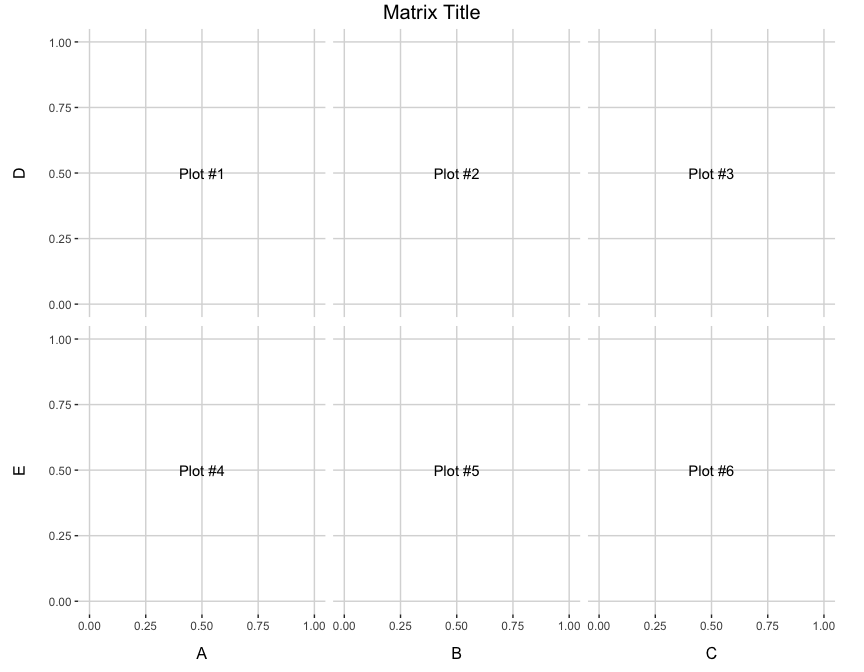``````# display plots in column order
pm <- ggmatrix(
plotList,
nrow = 2, ncol = 3,
xAxisLabels = c("A", "B", "C"),
yAxisLabels = c("D", "E"),
title = "Matrix Title",
byrow = FALSE
)
pm``````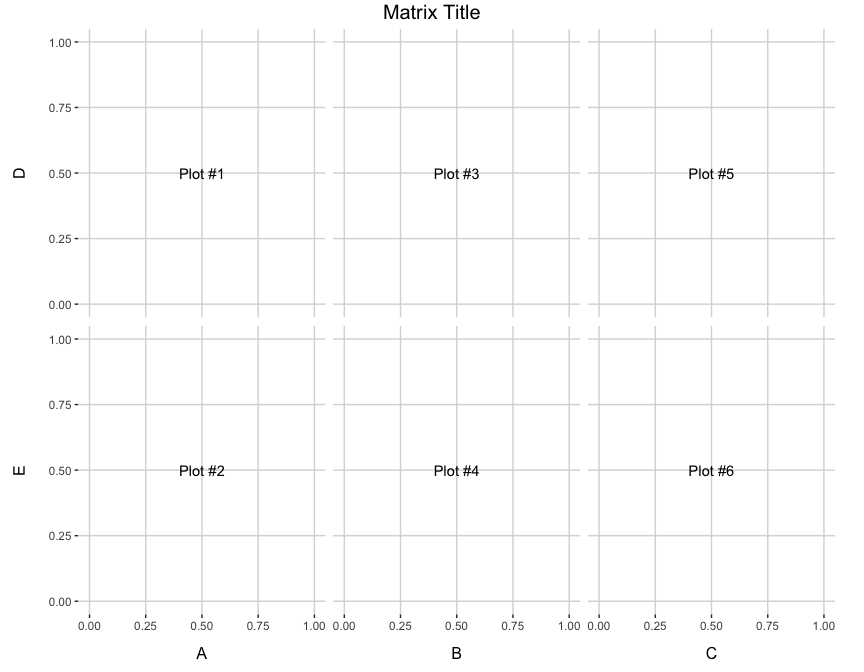## Matrix Subsetting

Individual plots may be retrieved from the plot matrix and can be placed in the plot matrix.

``````pm <- ggmatrix(
plotList,
nrow = 2, ncol = 3,
xAxisLabels = c("A", "B", "C"),
yAxisLabels = c("D", "E"),
title = "Matrix Title"
)
pm````````````p2 <- pm[1,2]
p3 <- pm[1,3]
p2``````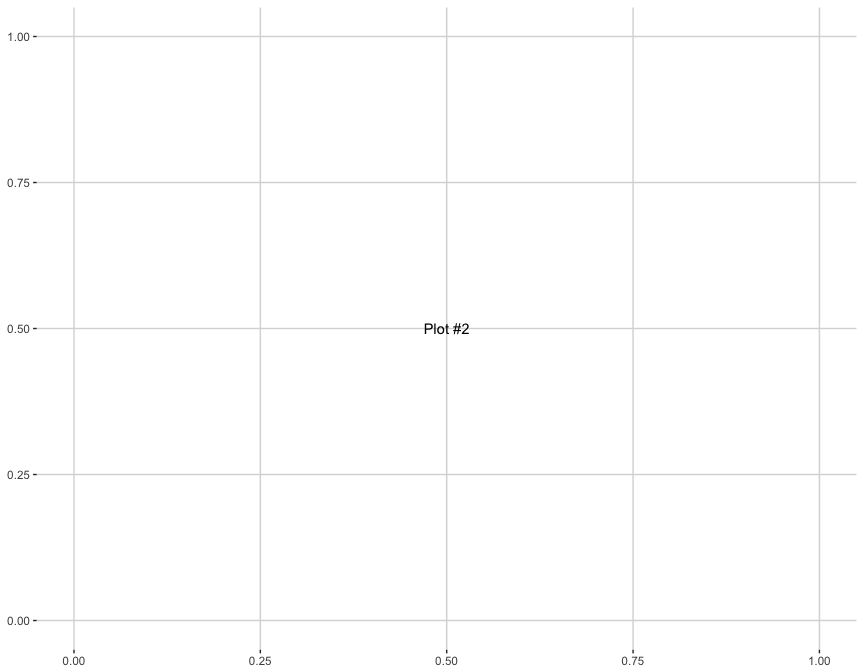``p3``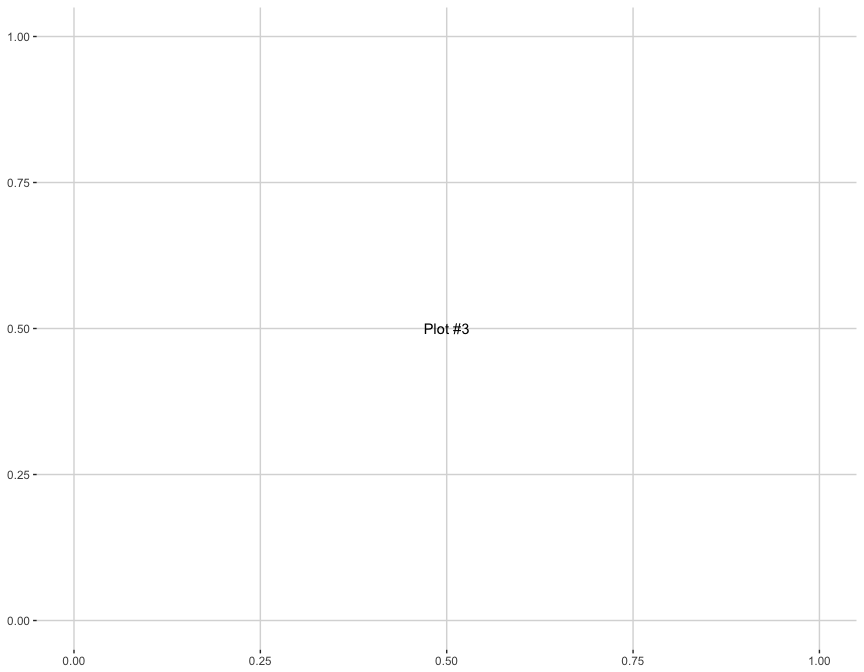``````pm[1,2] <- p3
pm[1,3] <- p2
pm``````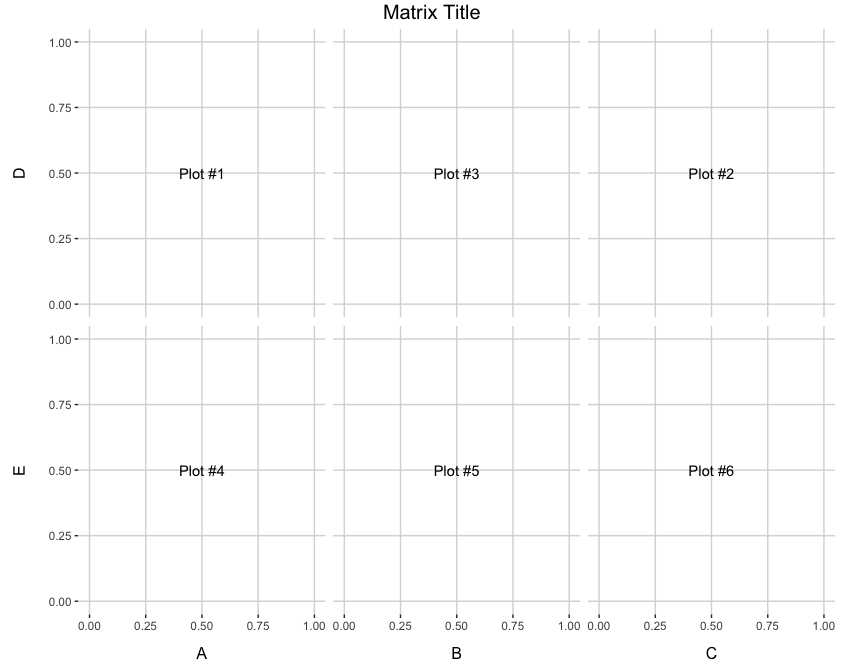## Themes

``````pm <- ggmatrix(
plotList,
nrow = 2, ncol = 3,
xAxisLabels = c("A", "B", "C"),
yAxisLabels = c("D", "E"),
title = "Matrix Title",
byrow = FALSE
)
pm <- pm + theme_bw()
pm``````